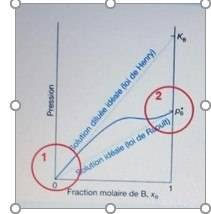# Henry's law applied to a solution of a solute in a solvent

• Chemistry
Homework Statement:
Let a solution of a solute B in a solvent A the vapour pressure is represented on the cluster below

A.The region in circle 1 accounts for the behavior of the solute in an ideally diluted solution
B.The vapour pressure of B is equal to Henry's constant in the case of an ideal solution?
C.Henry's constant is dimensional
D.Vapour pressure PB* corresponds to atmospheric pressure
E.The region in circle 2 accounts for the behaviour of the solvent in an ideally diluted solution.

I think it's B That's right?
Relevant Equations:
;Last edited by a moderator:

BvU
Homework Helper
I do not agree. Explain why you think so ...

Also: please explain why you think A, C, D, E are not correct....

##\ ##

i think
option b is incorrect because k= pB* i.e. the pressure of the pure body in an ideal solution
C. henry's constant has the dimensions of a pressure
D.la steam pressure of pB* corresponds to the pression of the pure body.
E. a solution ideally diluted follows Henry's law and in circle 2 we see raoult's law.

BvU
Homework Helper
option b is incorrect because k= pB* i.e. the pressure of the pure body in an ideal solution
I find your picture difficult to read, but it seems to me the top right symbol is ##K_B## and the straight line says 'Ideal dilute solution (Henry's law)'.

In region 1 the ##p(x_B)## follows the straight line closely, so there you have ##p = K_B \,x_B##.
(so not k= pB* !!)
In this case the constant ##K_B## has the dimension of pressure / mole fraction, i.e. it is not dimensionless.
(so doesn't that mean that answer C is correct too ? Unfortunately there are several forms of Henry's law -- also check here)

I can only assume that the ordinate is the partial pressure of B, although the axis title is 'Pressure'.

In summary: A seems correct, B definitely not, C seems correct too. D is nonsense and E can be argued correct as well: in that region component B can be considered to be 'the solvent'

I request a second opinion from @Chestermiller

##\ ##

Chestermiller
Mentor
I don’t think that E is correct.

•BvU